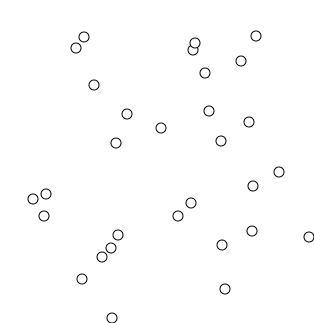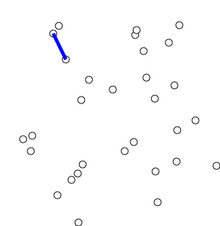# 最小生成树与并查集（leetcode684，685， 721）

## 最小生成树

### Kruskal算法

• 新建图G，G中拥有原图中相同的结点，但是没有边
• 将原图中所有边按照权值从小到大排序
• 从权值最小的边开始，如果这条边连接的两个节点不在一个连通分量中（也就是不形成环），则添加这条边到图G中
• 重复3，直至图G中所有的节点都在同一个连通分量中
•Kruskal算法是一种贪心算法，并且已经呗证明最终能够收敛到最好的结果。在实现Krusal算法时，则需要用到并查集这种数据结构来减小算法的时间复杂度，下面将详细介绍这种数据结构。

### Prime算法

Prime算法过程：

• 输入：顶点集合为 V V ，边集合为 E E
• 初始化： V n e w = n u l l ， E n e w = n u l l V_{new}=null， E_{new}=null
• 随机选择一个结点v加入到 V n e w V_{new}
• 重复下列操作，直到 V n e w = V V_{new}=V
1. 在集合 E E 中选取权值最小的边 ( u , v ) (u, v) ，其中 u u 为集合 V n e w V_{new} 中的元素，而 v v V − V n e w V-V_{new} 中的顶点；
2. 将 v v 加入到集合 V n e w V_{new} 中，将 ( u , v ) (u,v) 加入到边集合 E n e w E_{new} 中；
• 输出：使用集合 V n e w V_{new} E n e w E_{new} 来描述所得到的最小生成树
merge## 并查集

Kruskal算法中需要判断两个节点是否在同一个连通分量中，如何判断是否是一个连通分量呢？？也就判断是否加入新边之后，是否会和原来已经添加的边形成环路，并查集正是高效的实现了这个功能。

### 三个操作

• MakeSet是初始化操作，即为每一个node创建一个连通分量，且这个node为这个连通分量的代表，这里的连通分量的代表指的是当连通分量中有多个点中，需要从这些点中选出一个点来代表这个连通分量，而这个点也被称为这个连通分量的parent；
• Find是指找到这个点所属的连通分量的parent；
• Union是指将两个连通分量合并成一个连通分量，并选出代表这个连通分量的新的parent；

1. 给定一条边，为这两条边的两个顶点执行find操作，如果两个顶点的parent一样，那么就说明这两个点已经在同一个连通分量中，再添加就会导致闭环，不添加该边；
2. 当两个点的parent不同时，即两个点在不同的连通分量中，需要通过union操作将这两个连通分量连起来；
3. 重复1,2步操作直到所有的边遍历完；

### 具体题目

#### leetcode 684

684.Redundant Connection这道题目实际上就是要找到一个无向图中形成环路的最后那条边（输入保证了所有边会形成回路）

vector<int> findRedundantConnection(vector<vector<int>>& edges) {
vector<int> root(2000, 0);
for(int i=0; i<2000; i++)
root[i]=i;
vector<int> res;
for(auto edge : edges)
{
int a = edge;
int b = edge;
#find
while(root[a]!=a)
a = root[a];
while(root[b]!=b)
b = root[b];
#union
if(a==b)
res = edge;
else
root[a]=b;
}
return res;

}


#### path compression和union by rank

Path compression:

Union by rank:

int root;
int rank;
int find(int node)
{
int ans = node;
while(root[node]!=node)
node = root[node];
int r = node;
while(root[ans]!=r)
{
int tmp = ans;
ans = root[ans];
root[tmp] = r;
}
return r;
}
vector<int> findRedundantConnection(vector<vector<int>>& edges)
{

for(int i=0; i<1000; i++)
{
root[i]=i;
rank[i]=0;
}
vector<int> res;
for(auto edge : edges)
{
int a = edge;
int b = edge;
#find
int p1 = find(a);
int p2 = find(b);
#union
if(p1==p2)
res = edge;
else if(rank[p1]>rank[p2])
{
root[p2] = p1;
}
else if(rank[p1]<rank[p2])
{
root[p1] = p2;
}
else
{
rank[p2]+=1;
root[p1]=p2;
}
}
return res;
}


#### leecode 685

685. Redundant Connection ||从前面的无向图升级到了有向图，对应的要求从原来的仅要求不形成环路升级到在不形成环路的基础上，拓扑必须要是一棵合法树，也就是每个点只能有一个父节点，例如 [[2,1],[3,1]] 这两条边虽然没有形成环路，但是 1 有两个父亲节点（2和3），因此不是一棵合法的树。

#### leetcode 721

721. Accounts-merge该任务的任务是连接同一个account的email，这非非常适用于并查集来实现。为了将这些emails进行group，每个group需要有一个代表（父节点）。在最初， 每个email是其自己的代表。每个accont中的emails很自然的属于同一个group，应该被分配到相同的parent。选择每个account中的第一个email作为父节点。在其后进行find和union操作进行查找和更新父节点。

class Solution {
public:
vector<vector<string>> accountsMerge(vector<vector<string>>& acts) {
map<string, string> owner;
map<string, string> parents;
map<string, set<string>> unions;
for (int i = 0; i < acts.size(); i++) {
for (int j = 1; j < acts[i].size(); j++) {
parents[acts[i][j]] = acts[i][j];
owner[acts[i][j]] = acts[i];
}
}
for (int i = 0; i < acts.size(); i++) {
string p = find(acts[i], parents);
for (int j = 2; j < acts[i].size(); j++)
parents[find(acts[i][j], parents)] = p;
}
for (int i = 0; i < acts.size(); i++)
for (int j = 1; j < acts[i].size(); j++)
unions[find(acts[i][j], parents)].insert(acts[i][j]);

vector<vector<string>> res;
for (pair<string, set<string>> p : unions) {
vector<string> emails(p.second.begin(), p.second.end());
emails.insert(emails.begin(), owner[p.first]);
res.push_back(emails);
}
return res;
}
private:
string find(string s, map<string, string>& p) {
return p[s] == s ? s : find(p[s], p);
}
};



### 参考资料

08-0460605-102万+
07-241226
01-232635
04-24
08-02287
04-2514万+
04-27
11-1356